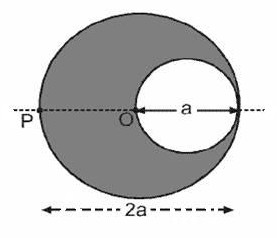## Friday, May 25, 2012

### IIT JEE 2012 Questions on Magnetic Field due to Infinitely Long Current Carrying Cylinders

“The world is a dangerous place, not because of those who do evil, but because of those who look on and do nothing.”
– Albert Einstein.

Today we will discuss two questions pertaining to the magnetic field produced by infinitely long current carrying cylinders. The first question is the usual single correct answer type multiple choice question. The second one is an integer answer type question, the answer to which is a single digit integer ranging from 0 to 9. Here are the questions with their solution.
(1) An infinitely long conducting cylinder with inner radius R/2 and outer radius R carries a uniform current density along its length. The magnitude of the magnetic field B‌ as a function of the radial distance r from the axis is best represented by‌‌Case (i): < R/2
Using Ampere’s circuital law we find that the magnetic fiel at points at distance r < R/2 is zero.
[We have ∫B.d = μ0I.
Since the current passing through the surface enclosed by the path of integration is zero, the value of B is zero at distance r < R/2]
‌‌Case (ii)
For distance r lying between R/2 and R (or, R/2 ≤ r < R) we have
∫B.d = μ0r2J π(R/2)2J] where J is the current density
r2J π(R/2)2J is the current passing through the surface enclosed by the path of integration in this case]
Or, B×2πr = μ0r2J π(R/2)2J]
This gives B = 0J/2)[r – (R2/4r)]
Case (iii)
For r R we have
B.d = μ0R2J π(R/2)2J]
Or, B×2πr = μ0R2J π(R/2)2J]
This gives B = 0J/2r)[R2 – (R2/4r)] = 3μ0JR2/8r
The graph shown in option (D) indicates the above three cases correctly.(2) A cylindrical cavity of diameter ‘a’ exists inside a cylinder of diameter 2a as shown in the figure. Both the cylinder and the cavity are infinitely long. A uniform current density J flows along the length. If the magnitude of the magnetic field at the point P is given by N μ0 aJ/12, then the value of N is
If the cylinder has no cavity, the magnetic flux density B1 at the point P is given by
∫B1.d = μ0πa2J
Therefore, B1×2πa = μ0πa2J
Or, B1 = μ0aJ/2
Since there exists a cavity, the current is reduced by π(a/2)2J and the magnetic field is reduced by B2.
The field B2 is given by
∫B2.d = μ0π(a/2)2J
Therefore, B2×2π(3a/2) = μ0π(a/2)2J
[The circular path of integration in this case has radius a+(a/2) = 3a/2]
Or, B2 = μ0aJ/12
The magnitude B of the magnetic field at the point P due to the actual conductor with the cavity is given by
B = B1 B2 =  μ0aJ/2μ0aJ/12
Or, B = 5μ0aJ/12
In the question the above field is given as Nμ0aJ/12
Therefore N = 5.